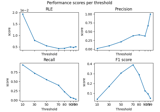# mne.simulation.metrics.peak_position_error#

mne.simulation.metrics.peak_position_error(stc_true, stc_est, src, threshold='50%', per_sample=True)[source]#

Compute the peak position error.

The peak position error measures the distance between the center-of-mass of the estimated and the true source.

$PPE = \| \dfrac{\sum_i|s_i|r_{i}}{\sum_i|s_i|} - r_{true}\|,$

where $$r_{true}$$ is a true dipole position, $$r_i$$ and $$|s_i|$$ denote respectively the position and amplitude of i-th dipole in source estimate.

Threshold is used on estimated source for focusing the metric to strong amplitudes and omitting the low-amplitude values.

Parameters:
stc_trueinstance of (Vol|Mixed)SourceEstimate

The source estimates containing correct values.

stc_estinstance of (Vol|Mixed)SourceEstimate

The source estimates containing estimated values e.g. obtained with a source imaging method.

srcinstance of SourceSpaces

The source space on which the source estimates are defined.

threshold

The threshold to apply to source estimates before computing the recall. If a string the threshold is a percentage and it should end with the percent character.

per_samplebool

If True the metric is computed for each sample separately. If False, the metric is spatio-temporal.

Returns:
metricfloat | array, shape (n_times,)

The metric. float if per_sample is False, else array with the values computed for each time point.

Notes

These metrics are documented in  and .

New in v1.2.

References

## Examples using mne.simulation.metrics.peak_position_error#Compare simulated and estimated source activity

Compare simulated and estimated source activity